# Thermal and economic evaluation of phase change material volume fraction for thermocline tank used in concentrating sola

Updated：2020-06-02 10:04Source：Applied Energy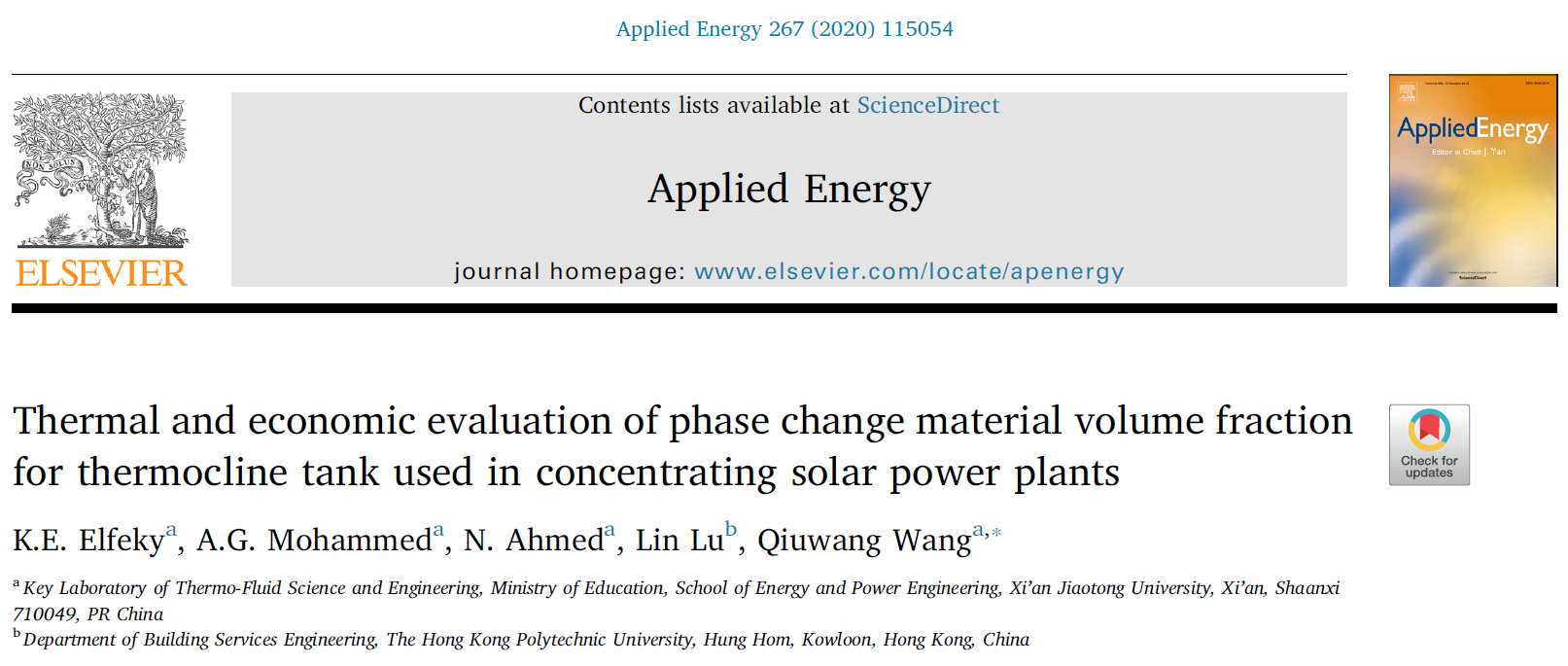Highlights

• The effect of PCM volume fraction for three-layers TES tank is investigated.

• A numerical model is used to define the temperature distribution of molten salt.

• The thermal/economic performance of the three-layers thermocline tank is evaluated.

• The bottom PCM layer with a higher VF has the lowest capacity cost of \$38/kWh.

Abstract

The current paper investigates the phase change material (PCM) volume fraction (VF) effect on the thermal and economic behavior of a three-layer thermocline thermal energy storage (TES) tank system which is used in concentrating solar power (CSP) plants. The one dimensional transient dispersion-concentric (D-C) scheme is applied to calculate the phase change inside each capsule. Using MATLAB software, the numerical model equations have been figured out, and the current numerical results have been verified. Four different scenarios have been created to investigate the effect of PCM-VF on the thermal behavior and economic feasibility of the TES tank. The results described that the overall efficiency and the cost of capacity for all cases in order from the case (1) to the case (4) are 80.77%, 64.32%, 73.43%, 85.58%, and \$45.37/kWh, \$60.49/kWh, \$46.28/kWh, \$38.58/kWh, respectively. Furthermore, case (4) demonstrates the storage capacity of 149 kWh/m3, which is 7.21%, 19.42%, and 15.78% higher than case (1), (2), and (3), respectively. In the comparative study, the results showed that case (4), which has higher VF for the bottom PCM layer, indicates that itis the most viable option of all the studied cases due to its best performance and relatively low cost.

Keywords：

Concentrating solar power plants

Volume fraction

Three-layers tank

Performance analysis

Cost analysis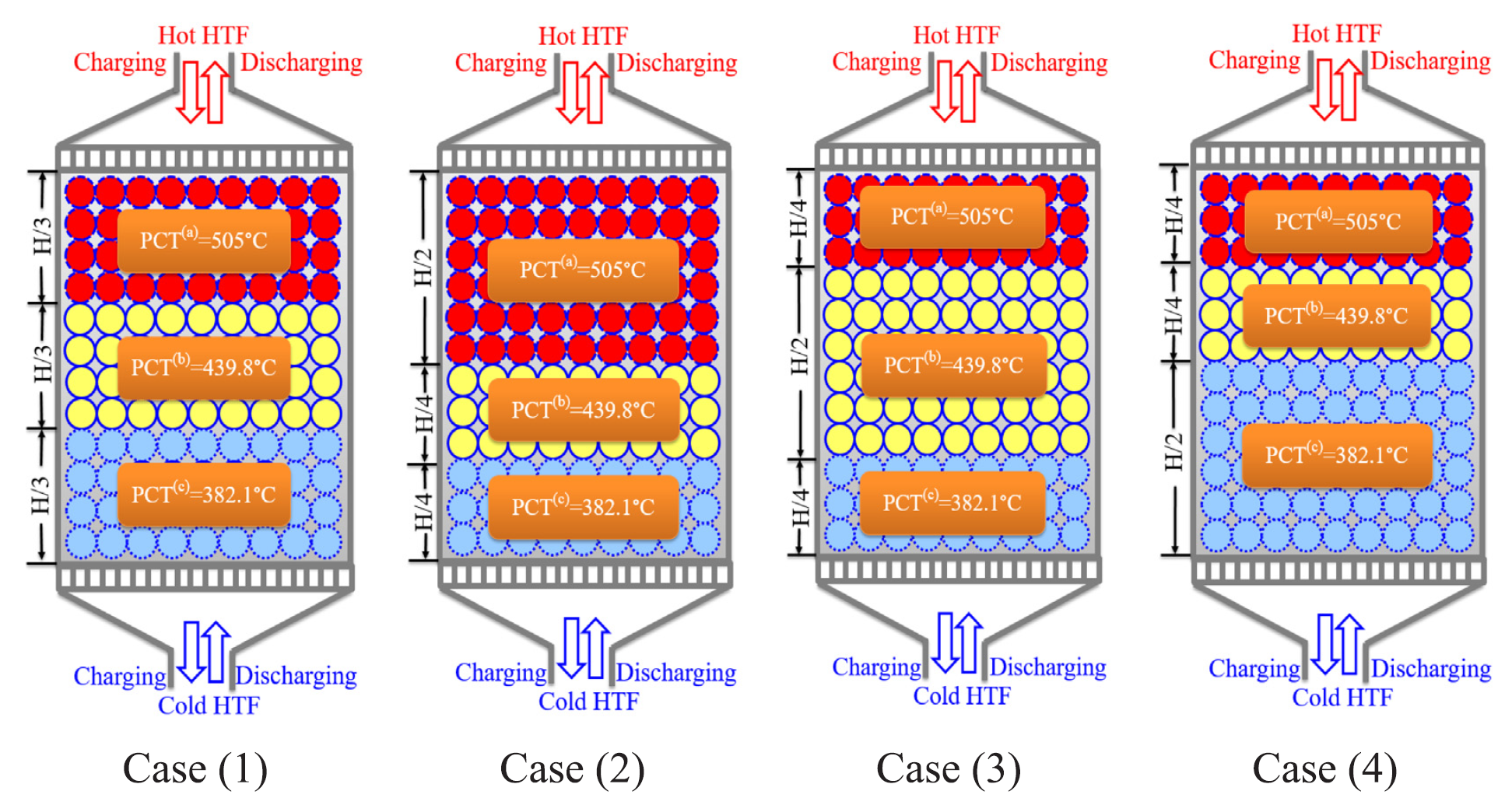Fig. 1. Schematic diagram showing the VF for each PCM layer for studied cases.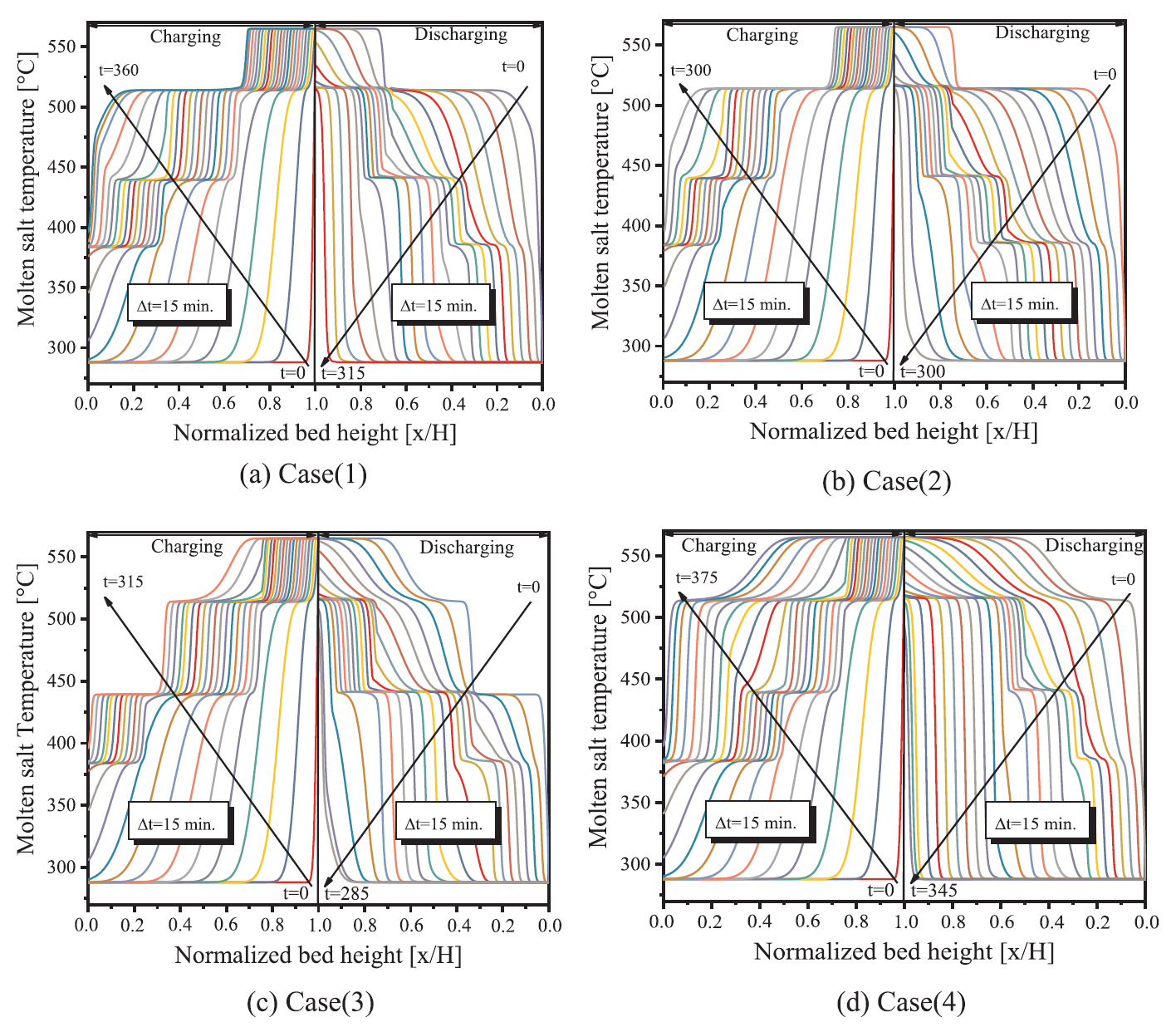Fig. 5. The axial distribution of temperature of the molten salt over the tank height at various time for charging and discharging cycles.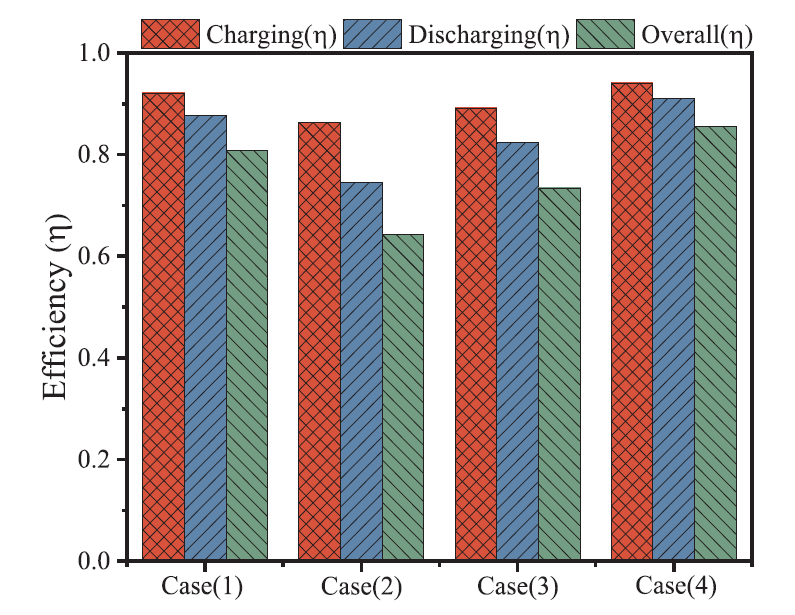Fig. 8. Charging, discharging, and overall efficiency of the TES thermocline tank.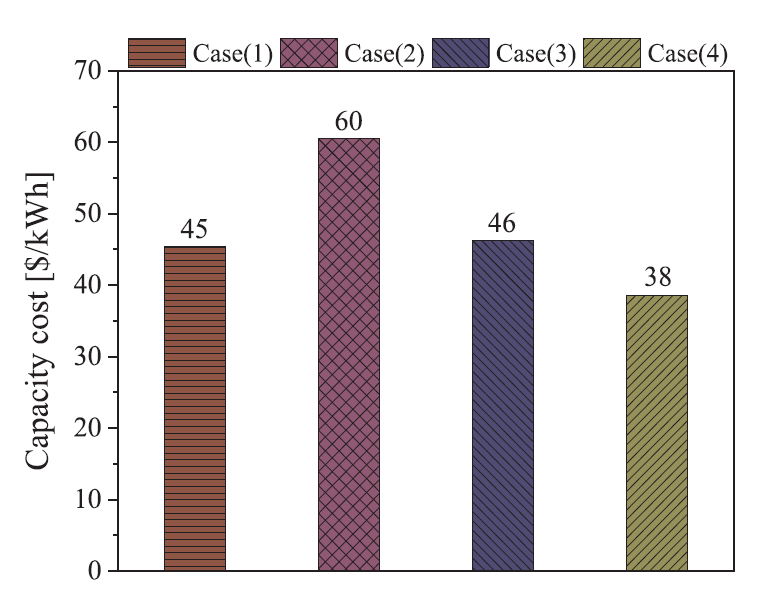Fig. 11. Showing the capacity cost (\$/kWh) for four different cases.

https://www.sciencedirect.com/science/article/pii/S0306261920305663